# 4.6 Scattering Self-Energies

The lowest-order electron-phonon self-energies are introduced in Section 3.6 and the steady-state form of these self-energies has been derived in Section 3.8.3. By transforming the self-energies (3.73) into mode-space one obtains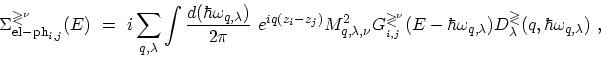(4.35)

where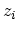is the position of some lattice point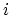along the CNT axis. Note that due to the one-dimensional nature of CNTs, the coordinate and wave-vector variables are all one-dimensional. In (4.35) only intra-subband scattering process are considered (see Section 2.6). To include inter-subband scattering processes the summation in (4.35) would have to run over the all subbands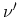with the electron-phonon matrix elements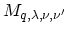.

The electron-phonon self-energies in the self-consistent BORN approximation are expressed in terms of the full electron and phonon GREEN's functions. One should therefore study the influence of the bare electron states on the phonons first, and then calculate the effect on the electrons of the renormalized phonon states . In this work we assume that the phonon renormalization can be neglected. By doing so we miss to capture a possible reduction of the phonon lifetime. The above considerations also appeal to the MIGDAL theorem  which states that the phonon-induced renormalization of the electron-phonon vertex (see Appendix F.2) scales with the ratio of the electron mass to the ion mass . Therefore, one can assume that the phonon bath is in thermal equilibrium so that the full phonon GREEN's function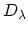can be replaced by the non-interacting GREEN's functions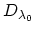from (D.16). As a result (4.35) can be written as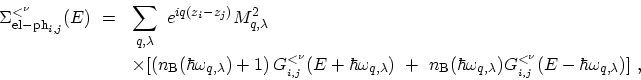(4.36)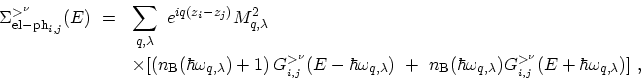(4.37)

where the first term on the right hand side is due to phonon emission and the second term due to phonon absorption. The summation over wave-vector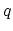in (4.36) and (4.37) can be generally transformed into an integral over the first BRILLOUIN zone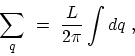(4.38)

where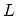is the normalization length and the limits of the integral are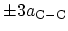which is the period of the carbon rings, see (4.3). To calculate electron-phonon self-energies the integral in (4.38) must be evaluated.

Subsections

M. Pourfath: Numerical Study of Quantum Transport in Carbon Nanotube-Based Transistors